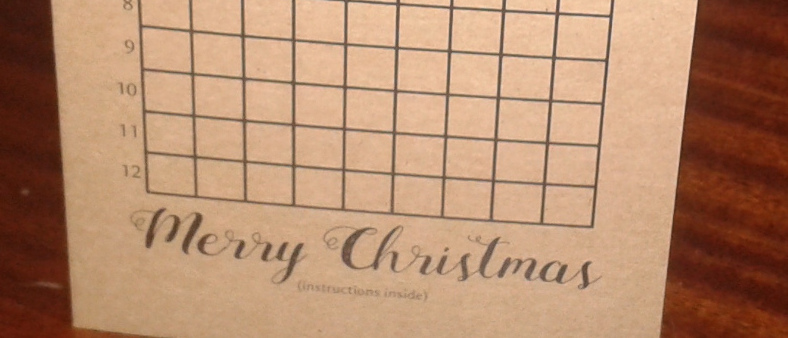# The Chalkdust Christmas card

This year’s Chalkdust puzzle Christmas cardThis post was part of the Chalkdust 2016 Advent Calendar.

Recently, some of you may have received a Chalkdust Christmas card. If not, it’s not because we hate you, it’s just that we couldn’t find your address… Unless we hate you, in which case it is because we hate you.

The card initially looks very boring: it is just a grid of squares with “Merry Christmas” written below it. Definitely NOT HOT… But there’s more. There’s a puzzle inside that leads you to add some colour to the squares to reveal a Christmassy picture.

Without giving any more away, here is the puzzle. If you’d like to give it to someone as a Christmas card (or just want to actually be able to colour it in), you can print and fold this lovely pdf.

### Christmas Card 2016

#### Instructions

1. Solve the puzzles below.
2. Convert the answers to base 3.
3. Write the answers in the boxes on the front cover.
4. Colour squares containing a 1 green. Colour squares containing a 2 red. Leave squares containing a 0 unshaded.

#### Puzzles

1. The square number larger than 1 whose square root is equal to the sum of its digits.
2. The smallest square number whose factors add up to a different square number.
3. The largest number that cannot be written in the form $23n+17m$, where $n$ and $m$ are positive integers (or 0).
4. Write down a three-digit number whose digits are decreasing. Write down the reverse of this number and find the difference. Add this difference to its reverse. What is the result?
5. The number of numbers between 0 and 10,000,000 that do not contain the digits 0, 1, 2, 3, 4, 5 or 6.
6. The lowest common multiple of 57 and 249.
7. The sum of all the odd numbers between 0 and 66.
8. One less than four times the 40th triangle number.
9. The number of factors of the number 2756×312.
10. In a book with 13,204 pages, what do the page numbers of the middle two pages add up to?
11. The number of off-diagonal elements in a 27×27 matrix.
12. The largest number, $k$, such that $27k/(27+k)$ is an integer.Matthew Scroggs is a postdoctoral researcher in the Department of Engineering at the University of Cambridge working on finite and boundary element methods. His website, mscroggs.co.uk, is full of maths.
@mscroggs    mscroggs.co.uk    + More articles by Matthew